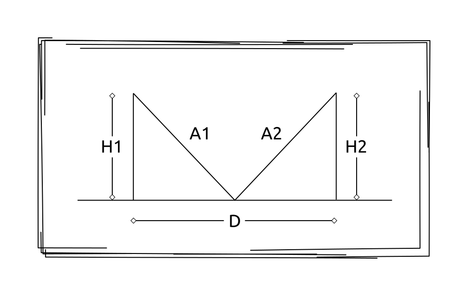# Lazy SupermenReplay of SCB-PA Inter Sc...
Limits 1s, 512 MB

There are two hills in Sonargaon. The heights of them are H1 and H2 meter. The distance between the two hills is D meter.Two supermen are on the top of the two hills. Now they want to meet each other on the ground. But there is a problem. Both of them want to cover as minimum distance as possible. Now your task is to find the distance each of them will cover.

Suppose one superman will go A1 distance and the other will go A2 distance. You have to minimize A1, A2 and the summation of A1 and A2.

Minimizing A1 and A2 means that the absolute difference |A1-A2| will be minimum.

## Input

The first line of input will contain one integer T (1 ≤ T ≤ 20), denoting the number of test cases.

Following T lines will contain 3 integers H1, H2 (0 ≤ H1, H2 ≤ 100000), and D (1 ≤ D ≤ 100000), denoting each test case.

## Output

For each test case, output a single line containing 2 integers A1 and A2. The results should be accurate at least four decimal places.

## Sample

InputOutput
3
24 15 100
100 250 34510
20 20 20
66.0529 41.2830
9860.5071 24651.2677
22.3607 22.3607

The hills are perpendicular to the ground.Math

### Submit